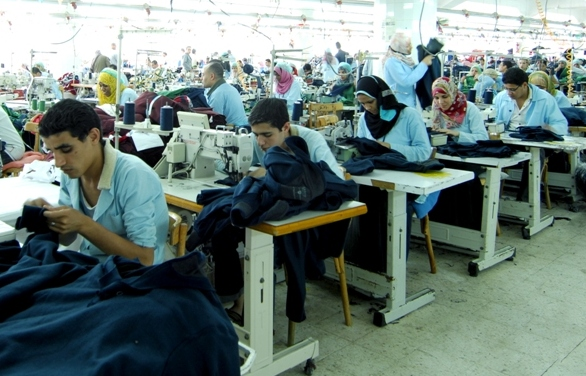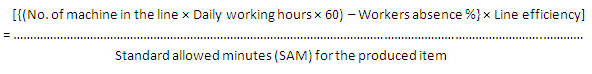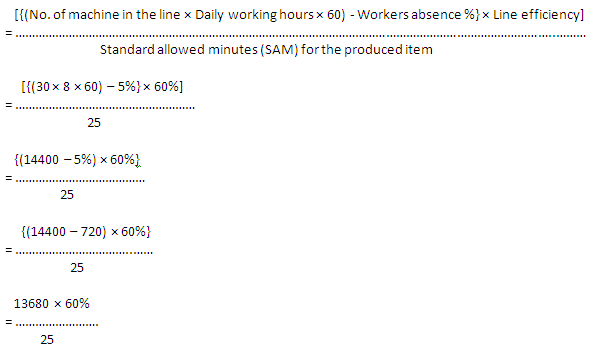# How to Calculate Sewing Line Capacity of an Apparel Industry?

By | October 28, 2015

### How to Calculate Sewing Line Capacity of an Apparel Industry?

Mayedul Islam
Merchandiser at Fashion Xpress Buying House.
Email: [email protected]

#### Introduction:

Sewing line capacity is an important matter in ready-made garments sector. By calculating sewing line capacity we can easily calculate the production capacity of any factory. As its importance, today I will present here the calculation method of sewing line’s capacity of an apparel industry.

You may follow Sewing Thread Consumption for Different StitchesSewing line capacity

#### Calculation method of sewing line capacity in apparel industry:

To calculate sewing lines capacity of a garment factory, an industrial engineer has needed the following information:

1. No. of sewing machines in the line,
2. Workers absence percentage of that line,
3. Daily working hours of that factory,
4. Line efficiency of that factory,
5. Standard allowed minutes (SAM) for the produced item.

Now, by using the below formula, an industrial engineer can easily calculate the sewing line’s capacity of the factory.

#### Sewing line capacity (In pcs),Example:

Suppose, in Abonty Fashions, a sewing line is running for producing full sleeve shirt with 30 machines at 8hrs working day, where sewing line’s efficiency is 60% and worker absent percentage is 5%.

Find out the sewing lines capacity for the above factory.

#### Solution:

Here,

No. of sewing machines in the line= 30,
Workers absence percentage of that line= 5%,
Daily working hours of that factory= 8,
Line efficiency of that factory= 60%,
Standard allowed minutes (SAM) for full sleeve shirt= 25.

So,

Sewing line capacity (In pcs),= 328.32

=328pcs

So, sewing line’s capacity per day for the above factory is 328pcs.

Speech from the writer:

If you read this article attentively then you can smartly answer the following question in the interview:

1. How to calculate sewing line’s capacity of a garment factory?
2. How to calculate sewing room capacity of a garment factory?
3. Describe the sewing section capacity calculation method for a garment factory.
4. Describe the sewing section capacity calculation method for an apparel industry.
5. Describe the sewing room capacity calculation method for an apparel industry.
6. Describe the sewing room capacity calculation method for a garment factory.

1.A Velliangiri A